# Density Estimation: Gaussian Mixture Models¶

Credits: Forked from PyCon 2015 Scikit-learn Tutorial by Jake VanderPlas

Here we'll explore Gaussian Mixture Models, which is an unsupervised clustering & density estimation technique.

In :
%matplotlib inline
import numpy as np
import matplotlib.pyplot as plt
from scipy import stats

# use seaborn plotting defaults
import seaborn as sns; sns.set()


## Introducing Gaussian Mixture Models¶

We previously saw an example of K-Means, which is a clustering algorithm which is most often fit using an expectation-maximization approach.

Here we'll consider an extension to this which is suitable for both clustering and density estimation.

For example, imagine we have some one-dimensional data in a particular distribution:

In :
np.random.seed(2)
x = np.concatenate([np.random.normal(0, 2, 2000),
np.random.normal(5, 5, 2000),
np.random.normal(3, 0.5, 600)])
plt.hist(x, 80, normed=True)
plt.xlim(-10, 20);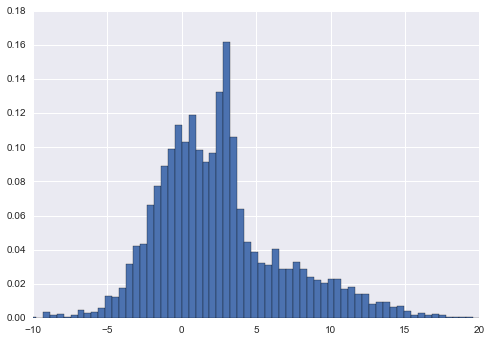Gaussian mixture models will allow us to approximate this density:

In :
from sklearn.mixture import GMM
clf = GMM(4, n_iter=500, random_state=3).fit(x)
xpdf = np.linspace(-10, 20, 1000)
density = np.exp(clf.score(xpdf))

plt.hist(x, 80, normed=True, alpha=0.5)
plt.plot(xpdf, density, '-r')
plt.xlim(-10, 20);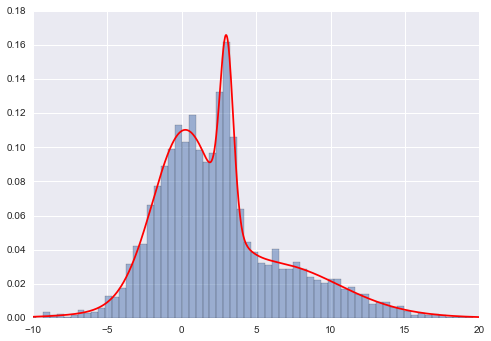Note that this density is fit using a mixture of Gaussians, which we can examine by looking at the means_, covars_, and weights_ attributes:

In :
clf.means_

Out:
array([[ 4.5601338 ],
[ 0.0861325 ],
[ 3.01890623],
[ 6.87627234]])
In :
clf.covars_

Out:
array([[ 30.34748627],
[  4.30521863],
[  0.19750802],
[ 14.78230876]])
In :
clf.weights_

Out:
array([ 0.27613209,  0.48308463,  0.11612442,  0.12465886])
In :
plt.hist(x, 80, normed=True, alpha=0.3)
plt.plot(xpdf, density, '-r')

for i in range(clf.n_components):
pdf = clf.weights_[i] * stats.norm(clf.means_[i, 0],
np.sqrt(clf.covars_[i, 0])).pdf(xpdf)
plt.fill(xpdf, pdf, facecolor='gray',
edgecolor='none', alpha=0.3)
plt.xlim(-10, 20);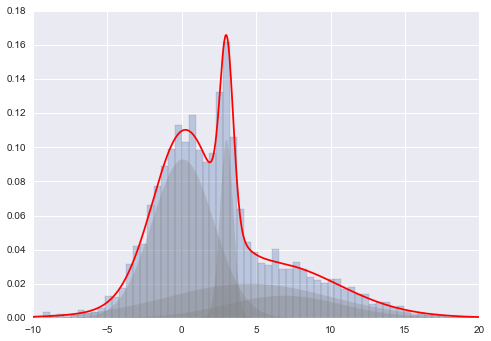These individual Gaussian distributions are fit using an expectation-maximization method, much as in K means, except that rather than explicit cluster assignment, the posterior probability is used to compute the weighted mean and covariance. Somewhat surprisingly, this algorithm provably converges to the optimum (though the optimum is not necessarily global).

## How many Gaussians?¶

Given a model, we can use one of several means to evaluate how well it fits the data. For example, there is the Aikaki Information Criterion (AIC) and the Bayesian Information Criterion (BIC)

In :
print(clf.bic(x))
print(clf.aic(x))

25696.4735953
25625.7016679


Let's take a look at these as a function of the number of gaussians:

In :
n_estimators = np.arange(1, 10)
clfs = [GMM(n, n_iter=1000).fit(x) for n in n_estimators]
bics = [clf.bic(x) for clf in clfs]
aics = [clf.aic(x) for clf in clfs]

plt.plot(n_estimators, bics, label='BIC')
plt.plot(n_estimators, aics, label='AIC')
plt.legend();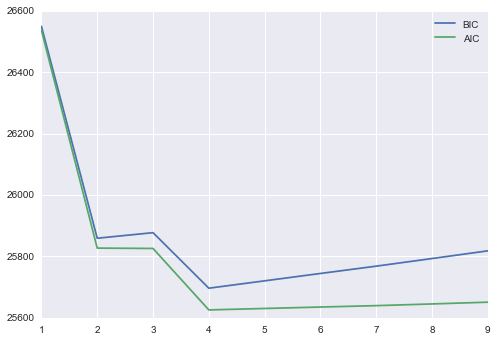It appears that for both the AIC and BIC, 4 components is preferred.

## Example: GMM For Outlier Detection¶

GMM is what's known as a Generative Model: it's a probabilistic model from which a dataset can be generated. One thing that generative models can be useful for is outlier detection: we can simply evaluate the likelihood of each point under the generative model; the points with a suitably low likelihood (where "suitable" is up to your own bias/variance preference) can be labeld outliers.

Let's take a look at this by defining a new dataset with some outliers:

In :
np.random.seed(0)

true_outliers = np.sort(np.random.randint(0, len(x), 20))
y = x.copy()
y[true_outliers] += 50 * np.random.randn(20)

In :
clf = GMM(4, n_iter=500, random_state=0).fit(y)
xpdf = np.linspace(-10, 20, 1000)
density_noise = np.exp(clf.score(xpdf))

plt.hist(y, 80, normed=True, alpha=0.5)
plt.plot(xpdf, density_noise, '-r')
#plt.xlim(-10, 20);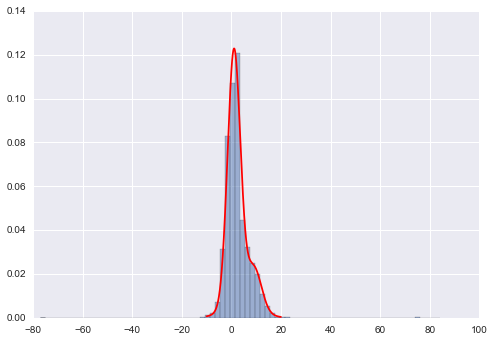Now let's evaluate the log-likelihood of each point under the model, and plot these as a function of y:

In :
log_likelihood = clf.score_samples(y)
plt.plot(y, log_likelihood, '.k');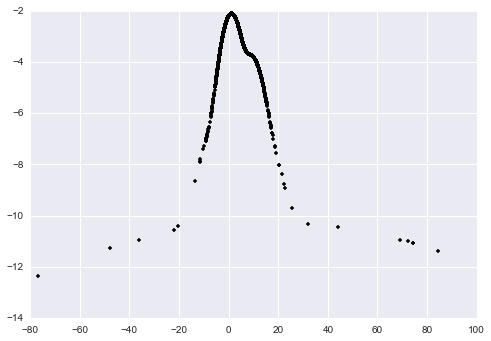In :
detected_outliers = np.where(log_likelihood < -9)

print("true outliers:")
print(true_outliers)
print("\ndetected outliers:")
print(detected_outliers)

true outliers:
[  99  537  705 1033 1653 1701 1871 2046 2135 2163 2222 2496 2599 2607 2732
2893 2897 3264 3468 4373]

detected outliers:
[ 537  705 1653 2046 2135 2163 2496 2732 2893 2897 3067 3468 4373]


The algorithm misses a few of these points, which is to be expected (some of the "outliers" actually land in the middle of the distribution!)

Here are the outliers that were missed:

In :
set(true_outliers) - set(detected_outliers)

Out:
{99, 1033, 1701, 1871, 2222, 2599, 2607, 3264}

And here are the non-outliers which were spuriously labeled outliers:

In :
set(detected_outliers) - set(true_outliers)

Out:
{3067}

Finally, we should note that although all of the above is done in one dimension, GMM does generalize to multiple dimensions, as we'll see in the breakout session.

## Other Density Estimators¶

The other main density estimator that you might find useful is Kernel Density Estimation, which is available via sklearn.neighbors.KernelDensity. In some ways, this can be thought of as a generalization of GMM where there is a gaussian placed at the location of every training point!

In :
from sklearn.neighbors import KernelDensity
kde = KernelDensity(0.15).fit(x[:, None])
density_kde = np.exp(kde.score_samples(xpdf[:, None]))

plt.hist(x, 80, normed=True, alpha=0.5)
plt.plot(xpdf, density, '-b', label='GMM')
plt.plot(xpdf, density_kde, '-r', label='KDE')
plt.xlim(-10, 20)
plt.legend();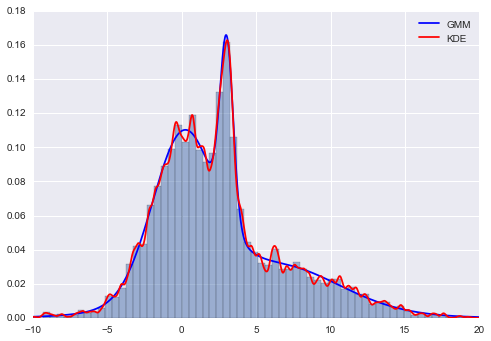All of these density estimators can be viewed as Generative models of the data: that is, that is, the model tells us how more data can be created which fits the model.